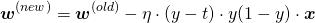# Gradient Descent for a Single Artificial Neuron

This article explores the gradient descent algorithm for a single artificial neuron. The activation function is set to the logistic function.

## Logistic Function

The logistic function is the most common kind of sigmoid function. It is defined as follows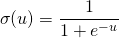## Properties

The logistic function has two primary good properties

1. The output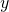is always between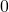and.
2. Unlike a unit step function,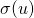is smooth and differentiable, making the derivation of update equation very easy.

##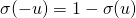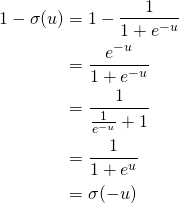##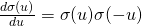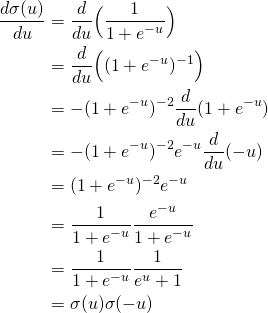## Notations

•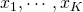are input values
•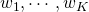are weights
•is a scalar output
•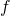is the activation function (also called decision/transfer function)
•is the label (gold standard)
•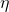is is the learning rate (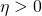)

The unit works in the following way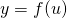where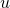is a scalar number, which is the net input of the neuron.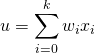In vector notation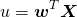To include a bias term, simply add an input dimension (e.g.,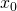) that is constant.

The error function (the training objective) is defined as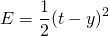Use stochastic gradient descent as the learning algorithm of this model – take the derivative of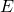with regard to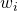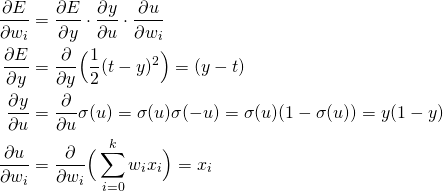Thus,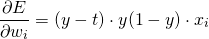Once the derivative is computed, simply apply stochastic gradient descent for all samples